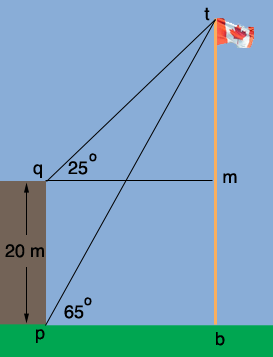SEARCH HOMEMath Central Quandaries & QueriesQuestion from paul, a student: A flag pole and a building stand on the same horizontal level. From the point p at the bottom of the building,the angle of elevation of the top t of the flag pole is 65 degrees. From the top q of the building the angle of elevation of the point t is 25 degrees.If the building is20 meters high. Calculate the distance ptHi Paul,

I drew a diagram and labeled a few more points.Look at the triangle tqp. From the information on the diagram you can determine the measures of the three angles in this triangle. Since you know the length of one of the sides you can use the Law of Sines to find the length of pt.

PennyMath Central is supported by the University of Regina and The Pacific Institute for the Mathematical Sciences.